Example Questions

← Previous 1 3 4

Example Question #1 : Least Common Multiple

What is the least common multiple of 4 and 6?

20

12

10

24

16

12

Explanation:

12 is the least common multiple of 4 and 6.

Example Question #2 : Least Common Multiple

What is the least common multiple of 8, 25, and 40?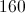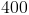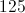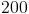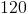Explanation:

Find the multiples of 40, and find the smallest one that is divisible by 8 and 25.

Example Question #3 : Least Common Multiple

If ab < 96 and b is a multiple of 4, what is the greatest possible value of a?

23.5

12

24

6

24

Explanation:

Since we want a to be as large as possible, we want b to be as small as possible.  The lowest possible value of b, then, is 4.  If b is 4, then we can solve for a by setting up the equation:

4(a) = 96

96/4 = 24

Example Question #4 : Least Common Multiple

What is the least common multiple of 20, 16, and 32?

320

4

5

160

160

Explanation:

The least comon multiple is the lowest multiple of all three numbers. 160 is 32x5, 16x10, and 8x20.

Example Question #5 : Least Common Multiple

What is the least common multiple ofand?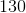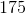There is no least common multiple becaues both numbers are prime.Explanation:

To find the least common multiple of two numbers, if they are both prime, simply multiply the numbers.

Thus we get that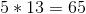We could also write out the multiples of each number and see what numbers they have in common. The least common multiple will be the common number that appears first in both sets.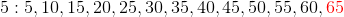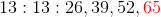Example Question #6 : Least Common Multiple

What is the least common multiple of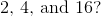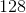Explanation:

To find the lowest common multiply, we need to find the smallest value which is a factor of each of the three numbers. We know it has to be even from the factor of two. If we look at the value, the lowest multiple of that number is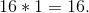Since the other two values are also divisible by this number, that is the lowest common multiple.

Example Question #7 : Least Common Multiple

A sliced cake can be equally split among 4, 5, or 6 people, with each person receiving the same number of slices. Which of the following answers represents a possible number of slices that the cake could have?

30

120

116

56

24

120

Explanation:

If you divide the answer choices by 4, 5, and 6, 120 is the only choice that divides evenly by all three numbers.

Example Question #1 : Greatest Common Factor

What is the greatest common factor of 49, 91, and 119?

7

3

9

1

7

Explanation:

The greatest common factor is the largest integer that divides without remainder into a set of integers. In this case, 1 and 7 are both common factors, but 7 is the greatest common factor.

Example Question #2 : Greatest Common Factor

What is the greatest common factor of 55, 165, and 220?

5

55

20

11

55

Explanation:

The greatest common factor is the largest factor that the given numbers have in common. 5 is a common factor, but it is not the greatest one; 11 and 20 are not common factors to the given numbers; however, 55 is a common factor of the given three numbers and is the greatest one at that.

Example Question #3 : Greatest Common Factor

What is the greatest common factor of 30, 90, and 120?

25

3

10

30

15